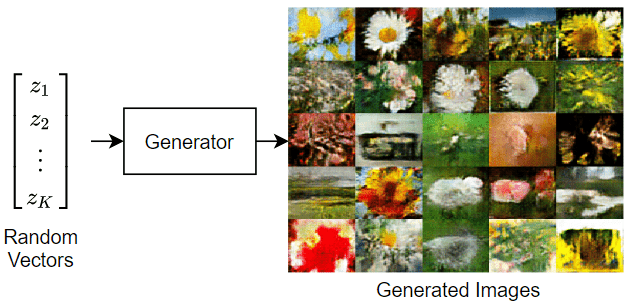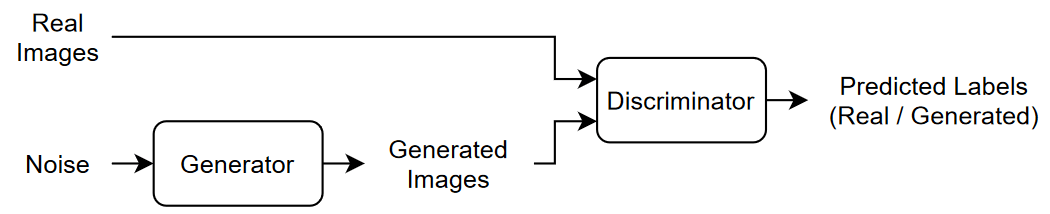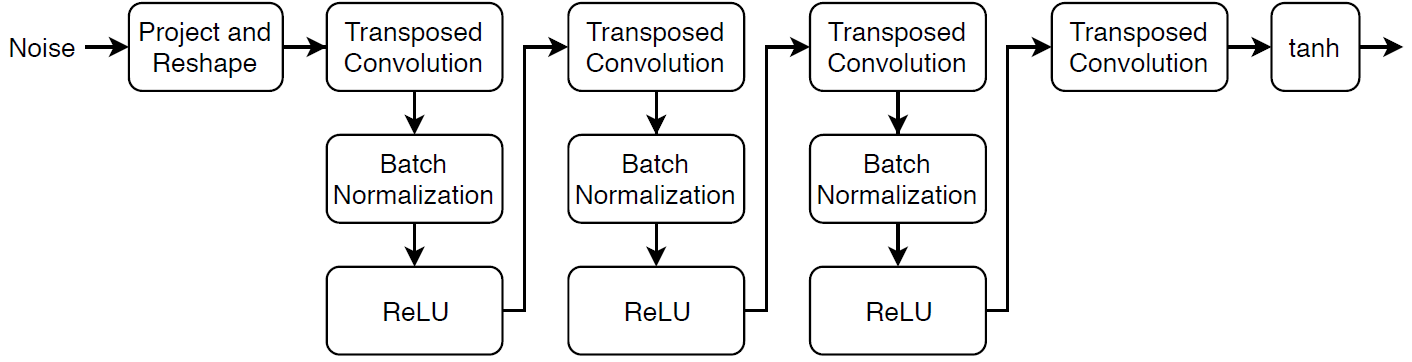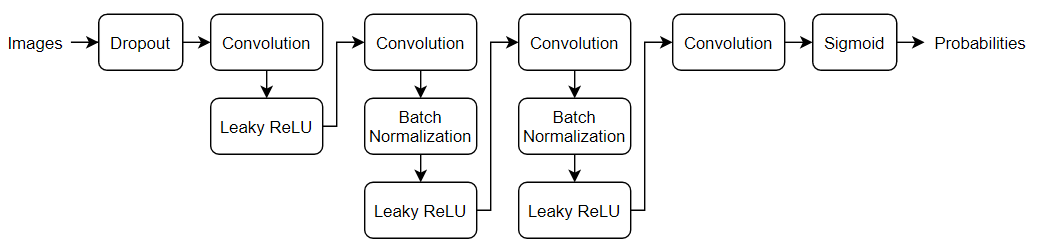# Train Generative Adversarial Network (GAN)

This example shows how to train a generative adversarial network to generate images.

A generative adversarial network (GAN) is a type of deep learning network that can generate data with similar characteristics as the input real data.

A GAN consists of two networks that train together:

1. Generator — Given a vector of random values (latent inputs) as input, this network generates data with the same structure as the training data.

2. Discriminator — Given batches of data containing observations from both the training data, and generated data from the generator, this network attempts to classify the observations as `"real"` or `"generated"`.To train a GAN, train both networks simultaneously to maximize the performance of both:

• Train the generator to generate data that "fools" the discriminator.

• Train the discriminator to distinguish between real and generated data.

To optimize the performance of the generator, maximize the loss of the discriminator when given generated data. That is, the objective of the generator is to generate data that the discriminator classifies as `"real"`.

To optimize the performance of the discriminator, minimize the loss of the discriminator when given batches of both real and generated data. That is, the objective of the discriminator is to not be "fooled" by the generator.

Ideally, these strategies result in a generator that generates convincingly realistic data and a discriminator that has learned strong feature representations that are characteristic of the training data.

```url = 'http://download.tensorflow.org/example_images/flower_photos.tgz'; downloadFolder = tempdir; filename = fullfile(downloadFolder,'flower_dataset.tgz'); imageFolder = fullfile(downloadFolder,'flower_photos'); if ~exist(imageFolder,'dir') disp('Downloading Flowers data set (218 MB)...') websave(filename,url); untar(filename,downloadFolder) end```

Create an image datastore containing the photos of the flowers.

```datasetFolder = fullfile(imageFolder); imds = imageDatastore(datasetFolder, ... 'IncludeSubfolders',true);```

Augment the data to include random horizontal flipping and resize the images to have size 64-by-64.

```augmenter = imageDataAugmenter('RandXReflection',true); augimds = augmentedImageDatastore([64 64],imds,'DataAugmentation',augmenter); ```

### Define Generator Network

Define the following network architecture, which generates images from random vectors of size 100.This network:

• Converts the random vectors of size 100 to 7-by-7-by-128 arrays using a project and reshape layer.

• Upscales the resulting arrays to 64-by-64-by-3 arrays using a series of transposed convolution layers with batch normalization and ReLU layers.

Define this network architecture as a layer graph and specify the following network properties.

• For the transposed convolution layers, specify 5-by-5 filters with a decreasing number of filters for each layer, a stride of 2, and cropping of the output on each edge.

• For the final transposed convolution layer, specify three 5-by-5 filters corresponding to the three RGB channels of the generated images, and the output size of the previous layer.

• At the end of the network, include a tanh layer.

To project and reshape the noise input, use the custom layer `projectAndReshapeLayer`, attached to this example as a supporting file. The `projectAndReshapeLayer` object upscales the input using a fully connected operation and reshapes the output to the specified size.

```filterSize = 5; numFilters = 64; numLatentInputs = 100; projectionSize = [4 4 512]; layersGenerator = [ featureInputLayer(numLatentInputs,'Name','in') projectAndReshapeLayer(projectionSize,numLatentInputs,'Name','proj'); transposedConv2dLayer(filterSize,4*numFilters,'Name','tconv1') batchNormalizationLayer('Name','bnorm1') reluLayer('Name','relu1') transposedConv2dLayer(filterSize,2*numFilters,'Stride',2,'Cropping','same','Name','tconv2') batchNormalizationLayer('Name','bnorm2') reluLayer('Name','relu2') transposedConv2dLayer(filterSize,numFilters,'Stride',2,'Cropping','same','Name','tconv3') batchNormalizationLayer('Name','bnorm3') reluLayer('Name','relu3') transposedConv2dLayer(filterSize,3,'Stride',2,'Cropping','same','Name','tconv4') tanhLayer('Name','tanh')]; lgraphGenerator = layerGraph(layersGenerator);```

To train the network with a custom training loop and enable automatic differentiation, convert the layer graph to a `dlnetwork` object.

`dlnetGenerator = dlnetwork(lgraphGenerator);`

### Define Discriminator Network

Define the following network, which classifies real and generated 64-by-64 images.Create a network that takes 64-by-64-by-3 images and returns a scalar prediction score using a series of convolution layers with batch normalization and leaky ReLU layers. Add noise to the input images using dropout.

• For the dropout layer, specify a dropout probability of 0.5.

• For the convolution layers, specify 5-by-5 filters with an increasing number of filters for each layer. Also specify a stride of 2 and padding of the output.

• For the leaky ReLU layers, specify a scale of 0.2.

• For the final layer, specify a convolutional layer with one 4-by-4 filter.

To output the probabilities in the range [0,1], use the `sigmoid` function in the `modelGradients` function, listed in the Model Gradients Function section of the example.

```dropoutProb = 0.5; numFilters = 64; scale = 0.2; inputSize = [64 64 3]; filterSize = 5; layersDiscriminator = [ imageInputLayer(inputSize,'Normalization','none','Name','in') dropoutLayer(dropoutProb,'Name','dropout') convolution2dLayer(filterSize,numFilters,'Stride',2,'Padding','same','Name','conv1') leakyReluLayer(scale,'Name','lrelu1') convolution2dLayer(filterSize,2*numFilters,'Stride',2,'Padding','same','Name','conv2') batchNormalizationLayer('Name','bn2') leakyReluLayer(scale,'Name','lrelu2') convolution2dLayer(filterSize,4*numFilters,'Stride',2,'Padding','same','Name','conv3') batchNormalizationLayer('Name','bn3') leakyReluLayer(scale,'Name','lrelu3') convolution2dLayer(filterSize,8*numFilters,'Stride',2,'Padding','same','Name','conv4') batchNormalizationLayer('Name','bn4') leakyReluLayer(scale,'Name','lrelu4') convolution2dLayer(4,1,'Name','conv5')]; lgraphDiscriminator = layerGraph(layersDiscriminator);```

To train the network with a custom training loop and enable automatic differentiation, convert the layer graph to a `dlnetwork` object.

`dlnetDiscriminator = dlnetwork(lgraphDiscriminator);`

### Define Model Gradients and Loss Functions

Create the function `modelGradients`, listed in the Model Gradients Function section of the example, which takes as input the generator and discriminator networks, a mini-batch of input data, an array of random values, and the flip factor, and returns the gradients of the loss with respect to the learnable parameters in the networks and the scores of the two networks.

### Specify Training Options

Train with a mini-batch size of 128 for 500 epochs. For larger data sets, you might not need to train for as many epochs.

```numEpochs = 500; miniBatchSize = 128;```

Specify the options for Adam optimization. For both networks, specify:

• A learning rate of 0.0002

• A gradient decay factor of 0.5

• A squared gradient decay factor of 0.999

```learnRate = 0.0002; gradientDecayFactor = 0.5; squaredGradientDecayFactor = 0.999;```

If the discriminator learns to discriminate between real and generated images too quickly, then the generator can fail to train. To better balance the learning of the discriminator and the generator, add noise to the real data by randomly flipping the labels.

Specify a `flipFactor` value of 0.3 to flip 30% of the real labels (15% of the total labels). Note that this does not impair the generator as all the generated images are still labeled correctly.

`flipFactor = 0.3;`

Display the generated validation images every 100 iterations.

`validationFrequency = 100;`

### Train Model

Use `minibatchqueue` to process and manage the mini-batches of images. For each mini-batch:

• Use the custom mini-batch preprocessing function `preprocessMiniBatch` (defined at the end of this example) to rescale the images in the range `[-1,1]`.

• Discard any partial mini-batches with less than 128 observations.

• Format the image data with the dimension labels `'SSCB'` (spatial, spatial, channel, batch). By default, the `minibatchqueue` object converts the data to `dlarray` objects with underlying type `single`.

• Train on a GPU if one is available. When the `'OutputEnvironment'` option of `minibatchqueue` is `"auto"`, `minibatchqueue` converts each output to a `gpuArray` if a GPU is available. Using a GPU requires Parallel Computing Toolbox™ and a CUDA® enabled NVIDIA® GPU with compute capability 3.0 or higher.

```augimds.MiniBatchSize = miniBatchSize; executionEnvironment = "auto"; mbq = minibatchqueue(augimds,... 'MiniBatchSize',miniBatchSize,... 'PartialMiniBatch','discard',... 'MiniBatchFcn', @preprocessMiniBatch,... 'MiniBatchFormat','SSCB',... 'OutputEnvironment',executionEnvironment);```

Train the model using a custom training loop. Loop over the training data and update the network parameters at each iteration. To monitor the training progress, display a batch of generated images using a held-out array of random values to input to the generator as well as a plot of the scores.

```trailingAvgGenerator = []; trailingAvgSqGenerator = []; trailingAvgDiscriminator = []; trailingAvgSqDiscriminator = [];```

To monitor the training progress, display a batch of generated images using a held-out batch of fixed random vectors fed into the generator and plot the network scores.

Create an array of held-out random values.

```numValidationImages = 25; ZValidation = randn(numLatentInputs,numValidationImages,'single');```

Convert the data to `dlarray` objects and specify the dimension labels `'CB'` (channel, batch).

`dlZValidation = dlarray(ZValidation,'CB');`

For GPU training, convert the data to `gpuArray` objects.

```if (executionEnvironment == "auto" && canUseGPU) || executionEnvironment == "gpu" dlZValidation = gpuArray(dlZValidation); end```

Initialize the training progress plots. Create a figure and resize it to have twice the width.

```f = figure; f.Position(3) = 2*f.Position(3);```

Create a subplot for the generated images and the network scores.

```imageAxes = subplot(1,2,1); scoreAxes = subplot(1,2,2);```

Initialize the animated lines for the scores plot.

```lineScoreGenerator = animatedline(scoreAxes,'Color',[0 0.447 0.741]); lineScoreDiscriminator = animatedline(scoreAxes, 'Color', [0.85 0.325 0.098]); legend('Generator','Discriminator'); ylim([0 1]) xlabel("Iteration") ylabel("Score") grid on```

Train the GAN. For each epoch, shuffle the datastore and loop over mini-batches of data.

For each mini-batch:

• Evaluate the model gradients using `dlfeval` and the `modelGradients` function.

• Update the network parameters using the `adamupdate` function.

• Plot the scores of the two networks.

• After every `validationFrequency` iterations, display a batch of generated images for a fixed held-out generator input.

Training can take some time to run.

```iteration = 0; start = tic; % Loop over epochs. for epoch = 1:numEpochs % Reset and shuffle datastore. shuffle(mbq); % Loop over mini-batches. while hasdata(mbq) iteration = iteration + 1; % Read mini-batch of data. dlX = next(mbq); % Generate latent inputs for the generator network. Convert to % dlarray and specify the dimension labels 'CB' (channel, batch). % If training on a GPU, then convert latent inputs to gpuArray. Z = randn(numLatentInputs,miniBatchSize,'single'); dlZ = dlarray(Z,'CB'); if (executionEnvironment == "auto" && canUseGPU) || executionEnvironment == "gpu" dlZ = gpuArray(dlZ); end % Evaluate the model gradients and the generator state using % dlfeval and the modelGradients function listed at the end of the % example. [gradientsGenerator, gradientsDiscriminator, stateGenerator, scoreGenerator, scoreDiscriminator] = ... dlfeval(@modelGradients, dlnetGenerator, dlnetDiscriminator, dlX, dlZ, flipFactor); dlnetGenerator.State = stateGenerator; % Update the discriminator network parameters. [dlnetDiscriminator,trailingAvgDiscriminator,trailingAvgSqDiscriminator] = ... adamupdate(dlnetDiscriminator, gradientsDiscriminator, ... trailingAvgDiscriminator, trailingAvgSqDiscriminator, iteration, ... learnRate, gradientDecayFactor, squaredGradientDecayFactor); % Update the generator network parameters. [dlnetGenerator,trailingAvgGenerator,trailingAvgSqGenerator] = ... adamupdate(dlnetGenerator, gradientsGenerator, ... trailingAvgGenerator, trailingAvgSqGenerator, iteration, ... learnRate, gradientDecayFactor, squaredGradientDecayFactor); % Every validationFrequency iterations, display batch of generated images using the % held-out generator input. if mod(iteration,validationFrequency) == 0 || iteration == 1 % Generate images using the held-out generator input. dlXGeneratedValidation = predict(dlnetGenerator,dlZValidation); % Tile and rescale the images in the range [0 1]. I = imtile(extractdata(dlXGeneratedValidation)); I = rescale(I); % Display the images. subplot(1,2,1); image(imageAxes,I) xticklabels([]); yticklabels([]); title("Generated Images"); end % Update the scores plot. subplot(1,2,2) addpoints(lineScoreGenerator,iteration,... double(gather(extractdata(scoreGenerator)))); addpoints(lineScoreDiscriminator,iteration,... double(gather(extractdata(scoreDiscriminator)))); % Update the title with training progress information. D = duration(0,0,toc(start),'Format','hh:mm:ss'); title(... "Epoch: " + epoch + ", " + ... "Iteration: " + iteration + ", " + ... "Elapsed: " + string(D)) drawnow end end```Here, the discriminator has learned a strong feature representation that identifies real images among generated images. In turn, the generator has learned a similarly strong feature representation that allows it to generate images similar to the training data.

The training plot shows the scores of the generator and discriminator networks. To learn more about how to interpret the network scores, see Monitor GAN Training Progress and Identify Common Failure Modes.

### Generate New Images

To generate new images, use the `predict` function on the generator with a `dlarray` object containing a batch of random vectors. To display the images together, use the `imtile` function and rescale the images using the `rescale` function.

Create a `dlarray` object containing a batch of 25 random vectors to input to the generator network.

```numObservations = 25; ZNew = randn(numLatentInputs,numObservations,'single'); dlZNew = dlarray(ZNew,'CB');```

To generate images using the GPU, also convert the data to `gpuArray` objects.

```if (executionEnvironment == "auto" && canUseGPU) || executionEnvironment == "gpu" dlZNew = gpuArray(dlZNew); end```

Generate new images using the `predict` function with the generator and the input data.

`dlXGeneratedNew = predict(dlnetGenerator,dlZNew);`

Display the images.

```I = imtile(extractdata(dlXGeneratedNew)); I = rescale(I); figure image(I) axis off title("Generated Images")```The function `modelGradients` takes as input the generator and discriminator `dlnetwork` objects `dlnetGenerator` and `dlnetDiscriminator`, a mini-batch of input data `dlX`, an array of random values `dlZ`, and the percentage of real labels to flip `flipFactor`, and returns the gradients of the loss with respect to the learnable parameters in the networks, the generator state, and the scores of the two networks. Because the discriminator output is not in the range [0,1], the `modelGradients` function applies the sigmoid function to convert it into probabilities.

```function [gradientsGenerator, gradientsDiscriminator, stateGenerator, scoreGenerator, scoreDiscriminator] = ... modelGradients(dlnetGenerator, dlnetDiscriminator, dlX, dlZ, flipFactor) % Calculate the predictions for real data with the discriminator network. dlYPred = forward(dlnetDiscriminator, dlX); % Calculate the predictions for generated data with the discriminator network. [dlXGenerated,stateGenerator] = forward(dlnetGenerator,dlZ); dlYPredGenerated = forward(dlnetDiscriminator, dlXGenerated); % Convert the discriminator outputs to probabilities. probGenerated = sigmoid(dlYPredGenerated); probReal = sigmoid(dlYPred); % Calculate the score of the discriminator. scoreDiscriminator = (mean(probReal) + mean(1-probGenerated)) / 2; % Calculate the score of the generator. scoreGenerator = mean(probGenerated); % Randomly flip a fraction of the labels of the real images. numObservations = size(probReal,4); idx = randperm(numObservations,floor(flipFactor * numObservations)); % Flip the labels. probReal(:,:,:,idx) = 1 - probReal(:,:,:,idx); % Calculate the GAN loss. [lossGenerator, lossDiscriminator] = ganLoss(probReal,probGenerated); % For each network, calculate the gradients with respect to the loss. gradientsGenerator = dlgradient(lossGenerator, dlnetGenerator.Learnables,'RetainData',true); gradientsDiscriminator = dlgradient(lossDiscriminator, dlnetDiscriminator.Learnables); end```

### GAN Loss Function and Scores

The objective of the generator is to generate data that the discriminator classifies as `"real"`. To maximize the probability that images from the generator are classified as real by the discriminator, minimize the negative log likelihood function.

Given the output $\mathit{Y}$ of the discriminator:

• $\stackrel{ˆ}{\mathit{Y}}=\sigma \left(\mathit{Y}\right)$ is the probability that the input image belongs to the class `"real"`.

• $1-\stackrel{ˆ}{\mathit{Y}}$ is the probability that the input image belongs to the class `"generated"`.

Note that the sigmoid operation $\sigma$ happens in the `modelGradients` function. The loss function for the generator is given by

`$\mathrm{lossGenerator}=-\mathrm{mean}\left(\mathrm{log}\left({\stackrel{ˆ}{\mathit{Y}}}_{\mathrm{Generated}}\right)\right),$`

where ${\underset{}{\overset{ˆ}{Y}}}_{Generated}$ contains the discriminator output probabilities for the generated images.

The objective of the discriminator is to not be "fooled" by the generator. To maximize the probability that the discriminator successfully discriminates between the real and generated images, minimize the sum of the corresponding negative log likelihood functions.

The loss function for the discriminator is given by

`$\mathrm{lossDiscriminator}=-\mathrm{mean}\left(\mathrm{log}\left({\stackrel{ˆ}{\mathit{Y}}}_{\mathrm{Real}}\right)\right)-\mathrm{mean}\left(\mathrm{log}\left(1-{\stackrel{ˆ}{\mathit{Y}}}_{\mathrm{Generated}}\right)\right),$`

where ${\underset{}{\overset{ˆ}{Y}}}_{Real}$ contains the discriminator output probabilities for the real images.

To measure on a scale from 0 to 1 how well the generator and discriminator achieve their respective goals, you can use the concept of score.

The generator score is the average of the probabilities corresponding to the discriminator output for the generated images:

`$\mathrm{scoreGenerator}=\mathrm{mean}\left({\stackrel{ˆ}{\mathit{Y}}}_{\mathrm{Generated}}\right).$`

The discriminator score is the average of the probabilities corresponding to the discriminator output for both the real and generated images:

`$\mathrm{scoreDiscriminator}=\frac{1}{2}\mathrm{mean}\left({\stackrel{ˆ}{\mathit{Y}}}_{\mathrm{Real}}\text{\hspace{0.17em}}\right)+\frac{1}{2}\mathrm{mean}\left(1-{\stackrel{ˆ}{\mathit{Y}}}_{\mathrm{Generated}}\right).$`

The score is inversely proportional to the loss but effectively contains the same information.

```function [lossGenerator, lossDiscriminator] = ganLoss(probReal,probGenerated) % Calculate the loss for the discriminator network. lossDiscriminator = -mean(log(probReal)) - mean(log(1-probGenerated)); % Calculate the loss for the generator network. lossGenerator = -mean(log(probGenerated)); end```

### Mini-Batch Preprocessing Function

The `preprocessMiniBatch` function preprocesses the data using the following steps:

1. Extract the image data from the incoming cell array and concatenate into a numeric array.

2. Rescale the images to be in the range `[-1,1]`.

```function X = preprocessMiniBatch(data) % Concatenate mini-batch X = cat(4,data{:}); % Rescale the images in the range [-1 1]. X = rescale(X,-1,1,'InputMin',0,'InputMax',255); end```

### References

2. Radford, Alec, Luke Metz, and Soumith Chintala. “Unsupervised Representation Learning with Deep Convolutional Generative Adversarial Networks.” Preprint, submitted November 19, 2015. http://arxiv.org/abs/1511.06434.